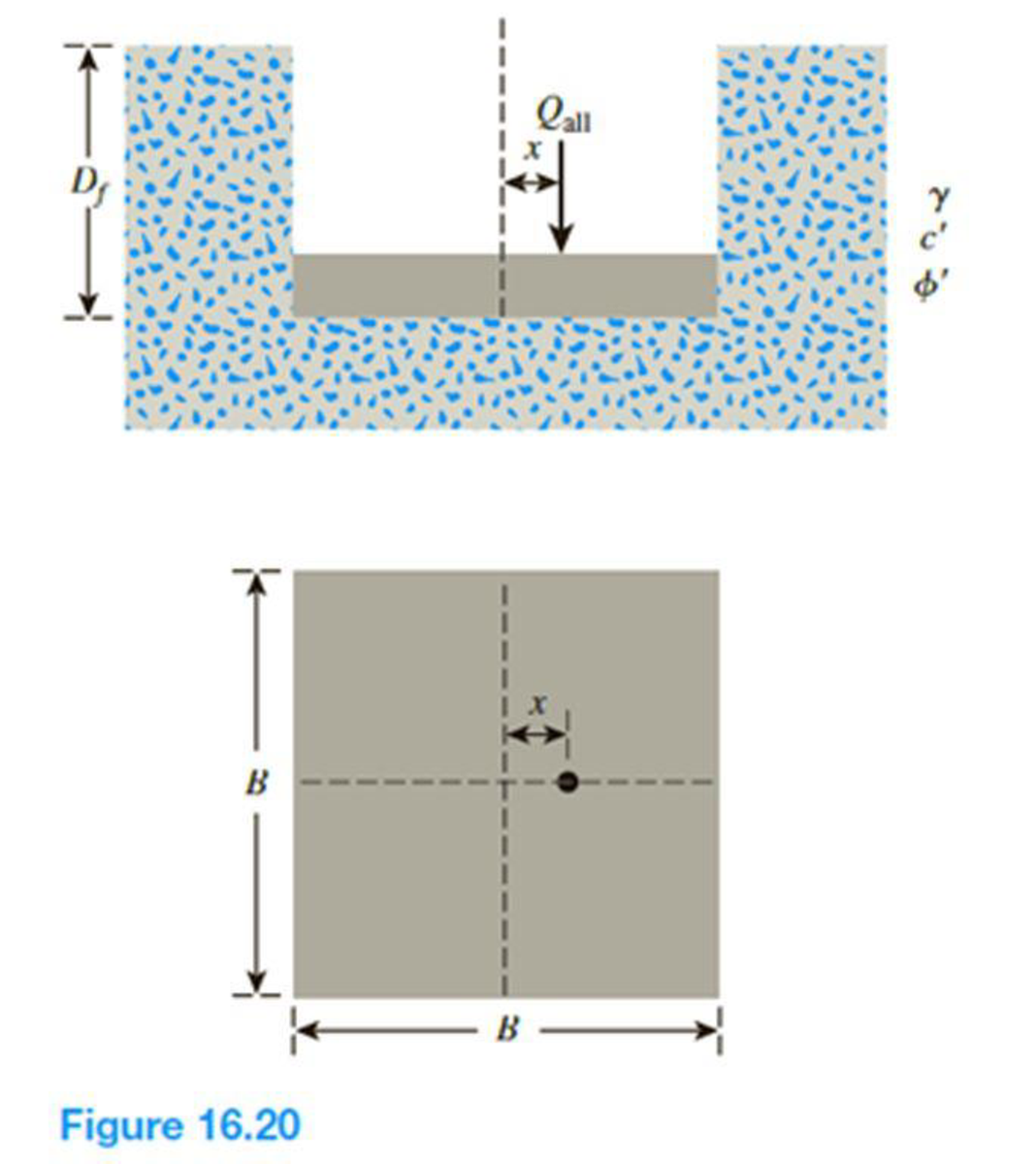Chapter 16, Problem 16.16PPrinciples of Geotechnical Enginee...

9th Edition
Braja M. Das + 1 other
ISBN: 9781305970939

Solutions

Chapter
SectionPrinciples of Geotechnical Enginee...

9th Edition
Braja M. Das + 1 other
ISBN: 9781305970939
Textbook Problem

A square footing on sand is subjected to an eccentric load as shown in Figure 16.20. Using Meyerhof’s effective area concept, determine the gross allowable load that the footing could carry with Fs = 4. Given: γ = 16 kN/m3, c′ = 0, ϕ′ = 29°, Df = 1.3 m, B = 1.75 m, and x = 0.25 m. Use Eqs. (16.32) through (16.42) for shape, depth, and inclination factors.To determine

Find the gross allowable load that the footing could carry using the effective area concept.

Explanation

Given information:

The unit weight of the soil γ is 16kN/m3.

The value of cohesion c is 0.

The soil friction angle ϕ is 29°.

The location of depth of footing base Df is 1.3 m.

The width of the footing B is 1.75 m.

The value of eccentricity x is 0.25 m.

The factor of safety value Fs is 4.

Calculation:

Determine the effective width of the footing using the relation.

B=B2x

Substitute 1.75 m for B and 0.25 for x.

B=1.752×0.25=1.25m

The effective length of the footing is taken as the larger value of B(1.75m) and B(1.25m).

Take L as 1.75 m.

Determine the shape factor λcs using the relation.

λcs=1+(BL)(NqNc)

Here, Nq and Nc is bearing-capacity factors.

Refer Table 16.2, “Bearing-capacity factors Nc, Nq, and Nγ” in the textbook.

For ϕ=29°;

The value of Nc is 27.86, Nq is 16.44, and Nγ is 19.34.

Substitute 1.25 m for B, 1.75 m for L, 16.44 for Nq, and 27.86 for Nc.

λcs=1+(1.251.75)(16.4427.86)=1.421

Determine the depth factor λcd using the relation.

λcd=1+0.4tan1(DfB)

Substitute 1.3 m for Df and 1.25 m for B.

λcd=1+0.4(1.31.25)=1.416

Determine the shape factor λqs using the relation.

λqs=1+(BL)tanϕ

Substitute 1.25 m for B, 1.75 m for L, and 29° for ϕ.

λqs=1+(1.251.75)tan29°=1.396

Determine the depth factor λqd using the relation.

λqd=1+2tanϕ(1sinϕ)2(DfB)

Substitute 29° for ϕ, 1.3 m for Df, and 1.25 m for B.

λqd=1+2tan29°(1sin29°)2(1

Still sussing out bartleby?

Check out a sample textbook solution.

See a sample solution

The Solution to Your Study Problems

Bartleby provides explanations to thousands of textbook problems written by our experts, many with advanced degrees!

Get Started

Compute the interest earned on the deposits made in Problem 20.1.

Engineering Fundamentals: An Introduction to Engineering (MindTap Course List)

What is a multicore processor?

Fundamentals of Information Systems

What is sparse data? Give an example.

Database Systems: Design, Implementation, & Management

What are some safe browsing techniques? (66)

Enhanced Discovering Computers 2017 (Shelly Cashman Series) (MindTap Course List)